# Topological semimetal in a fermionic optical lattice

## Abstract

Optical lattices have an important role in advancing our understandingof correlated quantum matter. The recent implementation of orbital degrees of freedom in chequerboard1,2 and hexagonal3 optical lattices opens up a new avenue towards discovering novel quantum states of matter that have no prior analogues in solid-state electronic materials. Here, we predict that an exotic topological semimetal emerges as a parity-protected gapless state in the orbital bands of a two-dimensional fermionic optical lattice. This new quantum state is characterized by a parabolic band-degeneracy point with Berry flux 2π, in sharp contrast to the π flux of Dirac points as in graphene. We show that the appearance of this topological liquid is universal for all lattices with D4 point-group symmetry, as long as orbitals with opposite parities hybridize strongly with each other and the band degeneracy is protected by odd parity. Turning on inter-particle repulsive interactions, the system undergoes a phase transition to a topological insulator whose experimental signature includes chiral gapless domain-wall modes, reminiscent of quantum Hall edge states.

## Main

The search for topological states of matter has been a focus of theoretical and experimental studies since the discovery of the quantum Hall effect4. This problem was brought to the forefront again recently by the theoretical prediction and experimental discovery of the time-reversal invariant Z2 topological insulators in semiconductors with strong spin–orbit couplings5,6,7,8,9,10,11. (For recent reviews see refs 12 and 13.) For non-interacting particles, the topological properties of insulators as well as topological superconductors have recently been classified on the basis of the anti-unitary symmetries of the systems14,15. However, this elegant topological classification does not apply to Fermi liquid (metal or semimetal) states owing to the existence of fermionic low-energy modes in gapless systems. In this paper, we shall show, however, that a novel type of topologically non-trivial semimetal unexpectedly arises as a universality class for arbitrary two-dimensional lattices with D4 point group symmetry owing to the mixing of orbitals of opposite parity. We believe that our discovery should be rather easily realizable in fermionic cold-atom optical lattices.

The physics of higher orbtials in optical lattices has recently emerged as an exciting new front in both theoretical16,17,18 and experimental (for example, early19,20,21,22 and recent1,2,3) studies. We specifically examine a model system that resembles the D4 symmetric double-well lattice reported in refs 1 and 2, but our conclusions apply generally to other lattices with the same point-group symmetry. Consider the optical lattice shown in Fig. 1a with the potential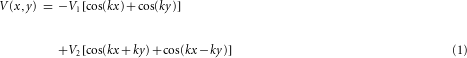Here, k=2π/a and a is the lattice constant. x and y are the coordinates in 2D configuration space. The parameters V 1 and V 2 are chosen to be positive. This optical lattice can be formed using a single chromatic light field following the experimental set-up shown in Fig. 1b for V 2/V 1≥1/2. For completeness, we will first consider the general situation with V 2/V 1≥0. Then, we will show that the parameter range of interest in our work is V 2/V 12/3>1/2, which can be realized using the proposed experimental set-up shown in Fig. 1b and is discussed in the Methods section.

For V 2=0, the V 1 term induces a square lattice with lattice constant a. As V 2 increases, the potential energy at the centre of a unit cell (with coordinates (0,0)) is increased whereas the potentials near the bond centres (with coordinates (±a/2,0) and (0,±a/2)) are reduced. For V 2>V 1/2, each unit cell contains potential minima located at (±a/2,0) and (0,±a/2), as shown in Fig. 1a.

We numerically solve the band structure of this lattice by means of plane-wave expansions and find that band degeneracy points appear between higher orbital bands at the Γ and M points (the centre and corner of the Brillouin zone). For the lowest four bands, as shown in Fig. 2, in the small- V 2 limit, the second and third bands cross at both Γ and M points. For larger V 2, there are still two band-degeneracy points for the lowest four bands, but now the second and third bands only cross at M, whereas the third and fourth bands become degenerate at Γ. For even larger V 2 (not shown), the first and second bands become degenerate at M, whereas the third and fourth bands touch at Γ. This large V 2 limit is dominated by the same physics as in the intermediate V 2 regime and, thus, we will only focus on the small and intermediate V 2 in this paper.Figure 2: The single-particle energy spectrum (measured in units of ER) for the lowest four bands and the topological structure near band-degeneracy points.

The band-degeneracy phenomenon described above is generic and stable. In fact, as shown in the Supplementary Information, for non-interacting particles these band-degeneracy points are topologically protected and remain stable when system parameters are tuned adiabatically, as long as the lattice point-group symmetry is maintained (although a band-degeneracy point may move from between the n and n+1 bands to the m and m+1 bands, as shown in the examples above). As detailed in the Methods section and the Supplementary Information, near the band-degeneracy point, a 2D vector field (hk) in momentum space can be defined using the Hamiltonian of the system. At momentum k, the length of this 2D vector (|hk|) gives (half of) the energy splitting between two energy bands. For the band-degeneracy points in our model, this vector field possesses a topological defect, a vortex with winding number 2. At the vortex core, the length of the vector vanishes (|hk|=0), indicating that the bandgap vanishes here (that is, a band-degeneracy point appears). It is this topological property that dictates the stability of the band degeneracy against any adiabatic deformation. From a mathematical point of view, this non-trivial topology can be described rigorously using the topological index of the Berry flux, which is 2π for this case.

In addition, the band-degeneracy point is also protected by the parity of the Bloch wavefunctions under space inversion. In fact, as shown in the Methods section, it turns out that all the essential physics of the topological semimetal can be understood within a simple tight-binding picture, without considering the full band-structure theory, and the key ingredient for this phenomenon is the mixing between the orbitals of opposite parity. In the particular model we consider here, the semimetal is formed by the hybridization between the d orbital and the two p (px and py) orbitals at each lattice site.

We now discuss the instability of the topological semimetal in the presence of interaction; the details are presented in Supplementary Information. We start with the tight-binding Hamiltonian and derive an effective low-energy theory around the Fermi point, which in this case is the degeneracy point of the third and fourth band (Fig. 2b). It turns out that this effective theory in the presence of interaction can be mapped onto a general theoretical model of d-wave symmetry which was analysed in refs 23, 24 by means of the renormalization-group technique. Therefore, by mapping the results back from that d-wave model, we obtain the universal property for the band-degeneracy point of the topological semimetal we present here. Below, we summarize the main results.

As the temperature is lowered below a critical value, Tc, the system undergoes a second-order phase transition, where TcWeα/N(0)V with N(0) the density of states at the chemical potential, V the interaction strength and Wthe bandwidth. The parameter α is a dimensionless constant whose value is determined by the band structure. In our model, the order parameter describing this low-temperature ordered phase is the z-component of the angular momentum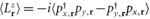, where px,r and py,r are the fermion annihilation operators of the px and py orbitals on site r. (This order parameter can be mapped to the order parameter ϕ in the general theory studied in ref. 23.)

In our system, the repulsive interaction can be reformulated as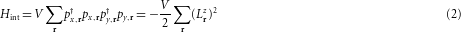where V >0 is the interaction strength. This interaction term favours a state with non-zero angular momentum 〈Lz〉≠0. In an ordinary metal or insulator (or graphene25), the formation of non-zero angular momentum costs kinetic energy, which usually dominates over the energy gain from interaction unless the interaction strength is very large. However, for the topological semimetal at low enough temperature, we find the energy cost for non-zero 〈Lz〉 from the kinetic part is always subleading compared with the energy gain from interaction. This results in the spontaneous generation of angular momentum, which is a key theoretical insight of our work.

From a symmetry point of view, this low-temperature phase spontaneously breaks the D4 point group symmetry down to C4, and also breaks the time-reversal symmetry. This symmetry-breaking pattern belongs to the Ising universality class, resulting in two degenerate ground states with opposite angular momentum.

As for the band structure, the band degeneracy at Γ is lifted in the symmetry-broken phase. (The degeneracy at M is also lifted. However, this is not relevant to our study as that degeneracy point is located far below the chemical potential.) Hence the topological semimetal becomes a fully gapped insulator in the presence of interaction, with the gap being VLz〉.

This insulator turns out to be topologically non-trivial, characterized by the non-trivial value of the topological index, known as the first Chern number. The Chern number for this state is 1, which indicates that this system shares the same topological properties as the quantum Hall state with filling 1. However, in contrast to the quantum Hall effect, where the non-trivial topological state is induced by a strong external magnetic field, here the same quantum topological state of matter originates from many-body effects in the absence of any external magnetic field. In general, states with non-zero Chern number in the absence of an external magnetic field are known as the anomalous quantum effect states, first proposed in a toy model on a honeycomb lattice by Haldane26. Recently, several different possible realizations of the Haldane model in cold gases have been discussed using lattice rotations27 or light-induced vector potentials28. In our predicted topological phase, however, interaction plays a decisive role, in sharp contrast to the non-interacting situation prevailing in the quantum Hall effect or anomalous quantum Hall effect. To the best of our knowledge, our work is the only theoretical prediction in the literature of an interaction-driven anomalous quantum Hall state.

Furthermore, if two spin components are both present in the atomic gases, the same interaction effect may lead to a time-reversal invariant Z2 topological insulator. This phenomenon can be partially understood as an interaction-driven 2D-version of HgTe. As pointed out in ref. 8, the combined effect of spin–orbit coupling and strain opens a gap at a 3D quadratic band-degeneracy point and leads to a 3D topological insulator. By contrast, in our 2D system, topological states arise purely because of many-body interaction effects.

To further demonstrate the topological nature of this insulating phase, we computed the band structure of this state on a cylinder, as shown in Fig. 3. Here, although the bulk modes are all gapped, there is a gapless topological chiral edge state on each of the two edges of the system, which is the direct signature of a topologically non-trivial insulator.

The phase transition being discussed in our work has a strong analogue in the Bardeen–Cooper–Schrieffer (BCS) theory of superconductivity. In particular, the two classes have similar scaling formula for the mean-field transition temperature (TCeα/N(0)V). However, the phase transition here breaks only a discrete symmetry (time reversal) and thus belongs to the Ising universality class. In 2D, the fluctuation effect is weak for an Ising transition and long-range order is sustained at finite temperature. In contrast, the BCS transition breaks the continuous U(1)symmetry and belongs to the X Y universality class. As a result, the BCS transition in 2D is a Kosterlitz–Thouless transition, whose transition temperature is strongly suppressed by phase fluctuations and is much lower than the mean-field prediction. Thus the transition temperature for our problem should be much higher than the BCS transition, if all other parameters (N(0), V, and so on) have the same value. Therefore, under equivalent conditions the phase transition predicted by us should be much easier to observe in 2D optical lattices than the corresponding BCS Kosterlitz–Thouless transition.

Beyond its theoretical significance, the topological semimetal state also has robust and unique experimental signatures. For example, the energy-band structure of the unique band-crossing degeneracy point can be detected directly using experimental techniques such as Bragg scattering29, as discussed in the Supplementary Information.

At low temperatures, the system remains a topological semimetal for attractive interactions but becomes an insulator for repulsive interactions. As both the values and the signs of interaction can be tuned in ultra-cold gases, this phase transition, between a compressible liquid and an incompressible insulator, can be studied experimentally by measuring the compressibility for different interactions. In addition, Bragg scattering can also be used to detect the insulating gap induced by the repulsive interactions. Because the low-temperature topological insulating state spontaneously breaks the time-reversal symmetry, any experimental measurements sensitive to the time-reversal symmetry, such as the Hall effect, can also be used to identify this phase.

The direct experimental evidence for a topological insulator is the gapless chiral edge state, which is a metallic state localized on the edge of a topologically non-trivial insulator. However, it is worthwhile to note that the sharp edge in the condensed matter system is absent in cold-atom gases. Owing to the existence of the slowly varying trap potential, one expects the density to decrease away from the centre of the trap. Therefore, the system is a liquid near the edge because of the low filling fraction. This liquid state from incommensurate filling will hybridize with the topological edge state, which makes the observation of the topological edge states challenging in atomic systems. This difficulty can be avoided if two domains of topological insulating phases with opposite angular momenta are induced. At the domain wall between these two areas, compressible chiral domain-wall states should exist. As this domain wall can be chosen to locate near the centre of the trap, far away from the trivial liquid state near the edge of the system, it should in principle provide a clean signature for the topological edge states. These domain-wall modes can also be detected using Bragg scattering, where one finds that the insulating gap is reduced to zero near the domain wall. In each real experimental system, owing to the finite number of particles on a particular optical lattice, the vanishing of the insulating gap at the domain wall is in fact prohibited by finite-size effects. For topological insulators, such finite-size effects have been systematically studied and the metallic edge states are found to be detectable even for a system of about ten particles30. An alternative experimental way of seeing the topological edge state would be to have a sharp trap boundary, as in a square-well potential, which would suppress the hybridization between the trivial liquid phase and the edge topological state. In such a square-well trap, the topological edge state should manifest itself directly.

## Methods

### Creation of the optical lattice.

In the experimental set-up shown in Fig. 1b of the Letter, by superimposing two monochromatic optical standing waves oscillating in phase, we implement the electric field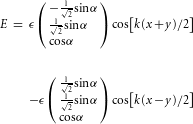The corresponding light-shift potential is U(x,y)=−χ|E(x,y)|2, with χ denoting the real part of the polarizability. It is straightforward to check that this potential is identical to the potential we propose in the main text, up to a trivial constant: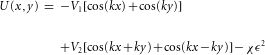with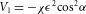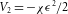By choosing blue detuning, that is, χ<0, we obtain V 1>0 and V 2>0. When the polarization direction, α, is changed, the ratio V 2/V 1can be tuned to any value above 1/2. For example, using fermionic potassium 40K with a principal fluorescence line at 767 nm, a standard green frequency-doubled Nd:YAG-laser (532 nm) would be a suitable light source for implementing the desired optical potential.

### Hybridization between orbitals of opposite parity.

It turns out that all the essential physics of the topological semimetal can be understood within a simple tight-binding picture and the key ingredient for this phenomenon is the mixing between orbitals with opposite parities under space inversion. Here, we outline the main procedures and results of the calculation for the mixing of parity-even $d x 2 − y 2$ and parity-odd px and py orbitals, and defer the details (for example, model Hamiltonian, band structure, and so on) to Section S-6 of the Supplementary Information. In this study, these three orbital bands are considered next to the chemical potential level and all other orbitals are assumed to be separated far from them (such that their effects can be dynamically ignored). When the mixing between the two types of orbitals is weak, the parity-odd orbitals form two bands, which cross each other at the Γ and M points, wheras the band formed by the parity-even orbitals shows no degeneracy (similar to the situation shown in Fig. 2a, which was obtained by numerical diagonalization). In contrast, as the mixing between different types of orbitals is enhanced, the three bands formed by these three orbitals hybridize together, and now the middle band crosses with both the other two bands, one at Γ and another at M, similar to Fig. 2b. In fact, the top three bands shown in Fig. 2 are mainly contributed by the px, py and d orbitals. In the Supplementary Information, a full comparison is provided between the band structure of the optical lattice model defined by the potential equation (1) and that of the effective three-orbital (px,py,$d x 2 − y 2$) tight-binding model.

### Instability under infinitesimal repulsion.

Using the conclusions from ref. 23, we found that under the renormalization group, the repulsive interaction shown in equation (2) is a marginally relevant perturbation and it is also the only relevant perturbation for spinless fermions with short-range interactions. Therefore, at low temperature, this interaction term dominates the low-energy physics and will stabilize a state with non-zero angular momentum 〈Lz〉≠0. This state is a topological insulator with Chern number 1, in agreement with the general study shown in ref. 23. This conclusion is further verified in Fig. 3, where we examined the mean-field single-particle spectrum for a cylindrical geometry and observed the gapless chiral edge states.

## References

1. 1

Wirth, G., Ölschläger, M. & Hemmerich, A. Evidence for orbital superfluidity in the p-band of a bipartite optical square lattice. Nature Phys. 7, 147–153 (2011).

2. 2

Ölschläger, M., Wirth, G. & Hemmerich, A. Unconventional superfluid order in the f band of a bipartite optical square lattice. Phys. Rev. Lett. 106, 015302 (2011).

3. 3

Soltan-Panahi, P., Lühmann, D-S., Struck, J., Windpassinger, P. & Sengstock, K. Quantum phase transition to unconventional multi-orbital superfluidity in optical lattices. Preprint at http://arxiv.org/abs/1104.3456 (2010).

4. 4

Nayak, C., Simon, S. H., Stern, A., Freedman, M. & Das Sarma, S. Non-Abelian anyons and topological quantum computation. Rev. Mod. Phys. 80, 1083–1159 (2008).

5. 5

Kane, C. & Mele, E. Z2 topological order and the quantum spin Hall effect. Phys. Rev. Lett. 95, 146802 (2005).

6. 6

Bernevig, B. A., Hughes, T. L. & Zhang, S-C. Quantum spin Hall effect and topological phase transition in HgTe quantum wells. Science 314, 1757–1761 (2006).

7. 7

Konig, M. et al. Quantum spin Hall insulator state in HgTe quantum wells. Science 318, 766–770 (2007).

8. 8

Fu, L. & Kane, C. L. Topological insulators with inversion symmetry. Phys. Rev. B 76, 045302 (2007).

9. 9

Moore, J. E. & Balents, L. Topological invariants of time-reversal-invariant band structures. Phys. Rev. B 75, 121306 (2007).

10. 10

Roy, R. Z2 classification of quantum spin Hall systems: An approach using time-reversal invariance. Phys. Rev. B 79, 195321 (2009).

11. 11

Hsieh, D. et al. A topological Dirac insulator in a quantum spin Hall phase. Nature 452, 970–974 (2008).

12. 12

Hasan, M. Z. & Kane, C. L. Colloquium: Topological insulators. Rev. Mod. Phys. 82, 3045–3067 (2010).

13. 13

Qi, X-L. & Zhang, S-C. Topological insulators and superconductors. Rev. Mod. Phys. 83, 1057–1110 (2011).

14. 14

Kitaev, A. Periodic table for topological insulators and superconductors. Preprint at http://arxiv.org/abs/0901.2686 (2009).

15. 15

Schnyder, A. P., Ryu, S., Furusaki, A. & Ludwig, A. W. W. Classification of topological insulators and superconductors in three spatial dimensions. Phys. Rev. B 78, 195125 (2008).

16. 16

Isacsson, A. & Girvin, S. M. Multi-flavor bosonic Hubbard models in the first excited Bloch band of an optical lattice. Phys. Rev. A 72, 053604 (2005).

17. 17

Liu, W. V. & Wu, C. Atomic matter of non-zero momentum Bose–Einstein condensation and orbital current order. Phys. Rev. A 74, 013607 (2006).

18. 18

Kuklov, A. B. Unconventional strongly interacting Bose–Einstein condensates in optical lattices. Phys. Rev. Lett. 97, 110405 (2006).

19. 19

Köhl, M., Moritz, H., Stöferle, T., Günter, K. & Esslinger, T. Fermionic atoms in a three dimensional optical lattice: Observing Fermi surfaces, dynamics, and interactions. Phys. Rev. Lett. 94, 080403 (2005).

20. 20

Browaeys, A. et al. Transport of atoms in a quantum conveyor belt. Phys. Rev. A 72, 053605 (2005).

21. 21

Lee, P. J. et al. Sublattice addressing and spin-dependent motion of atoms in a double-well lattice. Phys. Rev. Lett. 99, 020402 (2007).

22. 22

Müller, T., Fölling, S., Widera, A. & Bloch, I. State preparation and dynamics of ultracold atoms in higher lattice orbitals. Phys. Rev. Lett. 99, 200405 (2007).

23. 23

Sun, K., Yao, H., Fradkin, E. & Kivelson, S. A. Topological insulators and nematic phases from spontaneous symmetry breaking in 2d Fermi systems with a quadratic band crossing. Phys. Rev. Lett. 103, 046811 (2009).

24. 24

Sun, K. & Fradkin, E. Time-reversal symmetry breaking and spontaneous anomalous Hall effect in Fermi fluids. Phys. Rev. B 78, 245122 (2008).

25. 25

Das Sarma, S., Adam, S., Hwang, E. H. & Rossi, E. Electronic transport in two-dimensional graphene. Rev. Mod. Phys. 83, 407–470 (2011).

26. 26

Haldane, F. D. M. Model for a quantum Hall effect without Landau levels: Condensed-matter realization of the parity anomaly. Phys. Rev. Lett. 61, 2015–2018 (1988).

27. 27

Wu, C. Orbital analogue of the quantum anomalous Hall effect in p-band systems. Phys. Rev. Lett. 101, 186807 (2008).

28. 28

Stanescu, T. D., Galitski, V. & Das Sarma, S. Topological states in two-dimensional optical lattices. Phys. Rev. A 82, 013608 (2010).

29. 29

Ernst, P. T. et al. Probing superfluids in optical lattices by momentum-resolved Bragg spectroscopy. Nature Phys. 6, 56–61 (2010).

30. 30

Varney, C. N., Sun, K., Rigol, M. & Galitski, V. Interaction effects and quantum phase transitions in topological insulators. Phys. Rev. B 82, 115125 (2010).

## Acknowledgements

We appreciate the very helpful discussions with L. Fu, C. L. Kane and X-L. Qi. The work of K.S. and S.D.S. is supported by JQI-NSF-PFC, AFOSR-MURI, ARO-DARPA-OLE, and ARO-MURI. W.V.L. is supported by ARO (W911NF-07-1-0293 and 11-1-0230), ARO-DARPA-OLE (W911NF-07-1-0464) and the National Basic Research Program of China (Grant No 2012CB922101). A.H. acknlowledges support by DFG-SFB925. We thank the Kavli Institute for Theoretical Physics at UCSB for its hospitality where this research is supported in part by National Science Foundation Grant No. PHY05-51164.

## Author information

Authors

### Contributions

W.V.L., K.S., and S.D.S. planned the work. K.S. and W.V.L. carried out most of the calculations with input from S.D.S. A.H. provided the experimental protocol. All authors contributed to the writing of the manuscript.

### Corresponding author

Correspondence to W. Vincent Liu.

## Ethics declarations

### Competing interests

The authors declare no competing financial interests.

## Supplementary information

### Supplementary Information

Supplementary Information (PDF 836 kb)

## Rights and permissions

Reprints and Permissions

Sun, K., Liu, W., Hemmerich, A. et al. Topological semimetal in a fermionic optical lattice. Nature Phys 8, 67–70 (2012). https://doi.org/10.1038/nphys2134

• Accepted:

• Published:

• Issue Date:

• ### Bound states in two-dimensional Fermi systems with quadratic band touching

• Flávio L. N. Santos
• , Mônica A. Caracanhas
• , M. C. O. Aguiar
•  & Rodrigo G. Pereira

Physical Review B (2020)

• ### Non-Hermitian Floquet topological phases: Exceptional points, coalescent edge modes, and the skin effect

• Xizheng Zhang
•  & Jiangbin Gong

Physical Review B (2020)

• ### Hybrid Dispersion Dirac Semimetal and Hybrid Weyl Phases in Luttinger Semimetals: A Dynamical Approach

• Sayed Ali Akbar Ghorashi

Annalen der Physik (2020)

• ### Manifestation of a topological gapless phase in a two-dimensional chiral symmetric system through Loschmidt echo

• K. L. Zhang
•  & Z. Song

Physical Review B (2020)

• ### 4D spinless topological insulator in a periodic electric circuit

• Rui Yu
• , Y X Zhao
•  & Andreas P Schnyder

National Science Review (2020)

## Search